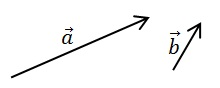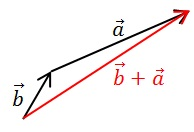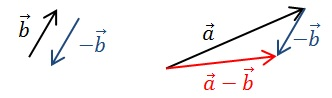# How to Add and Subtract Vectors

To add and subtract vectors that are quantities having magnitude and direction, there are special procedures. You can use the graphical method or the vector components to add and subtract vectors. This article explains both methods. First, let us see how to add vectors graphically.

## How to Add Vectors Graphically

Suppose we need to add the two vectors,$\vec{a}$ and$\vec{b}$Graphically, we place the “tail” of one of the vectors on the “nose” of the other vector. The resultant vector, i.e. the vector$\vec{a}+\vec{b}$, is the vector that goes from the tail of the first vector to the nose of the last vector. Note that we can repeat this procedure to add any number of vectors. In practice, to do this, one may need to make a scale diagram of the vectors on a paper.Vector addition is commutative, i. e.$\vec{a}+\vec{b}=\vec{b}+\vec{a}$. Graphically, we can show this by starting with$\vec{b}$ and then adding$\vec{a}$ to it. Since the resultant vector in both cases have the same size and direction, the resultant vectors are equal. This shows that the order in which vectors are added does not matter.Also, vector addition is associative, i.e.$\vec{a}+(\vec{b}+\vec{c})=(\vec{a}+\vec{b})+\vec{c}$. This means that the order in which vectors are grouped during addition does not matter.

## How to Add Vectors Using Components

Suppose the vector$\vec{a}=(a_x, a_y, a_z)$ and the vector$\vec{b}=(b_x, b_y, b_z)$. Then, the vector$\vec{a}+\vec{b}=(a_x+b_x, a_y+b_y, a_z+b_z)$.

Example

Two forces$\vec{F_1}$ and$\vec{F_2}$ act on a body. The forces are given by the vectors$\vec{F_1} = (2, 8, -5) \textup{N}$ and$\vec{F_2} = (-1, 3, 3) \textup{N}$. Find the resultant force$F_R$.$F_R = (2+(-1), 8+3, (-5)+3) = (1, 11, -2) \textup{N}$.

Similarly, subtracting vectors involves adding the negative of a vector.

## Negative of a Vector

For a given vector$\vec{p}$, the negative vector$\vec{-p}$ has the same magnitude as$\vec{p}$ but points in the opposite direction.## How to Subtract Vectors Graphically

Subtracting a vector is equivalent to adding the negative vector. i.e.$\vec{a}-\vec{b} = \vec{a}+(-\vec{b})$. Graphically, we can show this as follows:

We need to subtract$\vec{b}$ from$\vec{a}$.We identify the negative vector of$\vec{b}$. Then,$\vec{a}-\vec{b} = \vec{a}+(-\vec{b})$:## How to Subtract Vectors Using Components

Suppose the vector$\vec{a}=(a_x, a_y, a_z)$ and the vector$\vec{b}=(b_x, b_y, b_z)$. Then, the vector$\vec{a}-\vec{b}=(a_x-b_x, a_y-b_y, a_z-b_z)$.

For example, the displacement of a particle can be found by subtracting two position vectors. For worked example, see the article on displacement.

## How to Multiply Vectors